# A box

A box contains 6 red balls, 5 blue, and 4 white balls. Find the probability of drawing a) red ball b) non-white ball.

a =  0.4
b =  0.7333

### Step-by-step explanation:

$a=\frac{6}{6+5+4}=\frac{2}{5}=0.4$
$b=\frac{6+5}{6+5+4}=\frac{11}{15}=0.7333$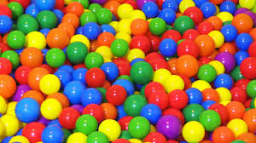Did you find an error or inaccuracy? Feel free to write us. Thank you!Tips to related online calculators
Would you like to compute count of combinations?

## Related math problems and questions:

• Components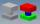In the box are 8 white, 4 blue and 2 red components. What is the probability that we pull one white, one blue and one red component without returning?
• BallsThe urn is 8 white and 6 black balls. We pull 4 randomly balls. What is the probability that among them will be two white?
• A bagA bag contains 9 blue marbles and 1 green marble. What is the probability of drawing a blue marble followed by a green marble, without replacing the first marble before drawing the second marble.
• Statistics quizFill in the missing word 1. in a data set, the mean, median and mode are measured of ________________ 2. "The manipulation of variables under controlled conditions"is the data collection method known as______________ 3. in a normal distribution, the area
• Red ballsIn the bag there are 3 red, 12 blue and 8 green balls. How many red balls we must be attached to the bag if we want the probability of pulling out the red balls was 20%?
• White and black ballsThere are 7 white and 3 black balls in an opaque pocket. The balls are the same size. a) Randomly pull out one ball. What is the probability that it will be white? We pull out one ball, see what color it is and return it to the pocket. Then we pull out th
• Round destiny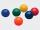There are 5 white and 10 red balls in the destiny. 4 balls will be drawn at random. What is the probability of the event "at least 2 spheres are white"?
• BallsFrom the urn in which are 7 white balls and 17 red, gradually drag 3-times without replacement. What is the probability that pulls balls are in order: red red red?
• Three colors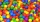Find the probability that 3 balls of the same color will be drawn from fate with 10 white, 10 red, and 10 blue balls.
• Chess piecesThere are 5 black chess pieces in the box. How many white pieces should we add to this box so that the probability of pulling out a black piece is 1: 4?
• Balls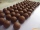From the bag with numbered balls (numbers 1,2,3,. ..20) we pick one ball. What is the probability of choosing a number containing 1?
• PC disksPeter has 45 disks in three colors. One-fifth of the disks are blue, red are twice more than the white. How much is blue, red and white disks?
• Screws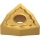The box contains 8 iron, 6 brass, and 4 titanium screws. What is the probability that the randomly selected screw will not be brass?
• Green - Red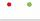We have 5 bags. Each consist one green and 2 red balls. From each we pull just one ball. What is the probability that we doesn't pull any green ball?
• Karolína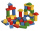Karolína chose 5 bodies from the kit - white, blue and gray cubes, a blue cylinder and a white triangular prism. How many different roof towers can be built one by one if all the blue bodies (cube and cylinder) are not placed on top of each other?
• There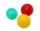There are two numbers on the screen - one in the blue and the other in the red box. In the beginning, both numbers are the same. With each beep, both numbers increase - by 1 in the blue field and by 3 in the red field. At one point, the number 49 appears
• Boats 2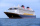Three quarters of the boats in the marina are white 4/7 of the remaining boats are blue, rest are red if there are 9 red boats. How many boats are in the marina?